DIFFUSICONE - EIGHT   and   UL-HC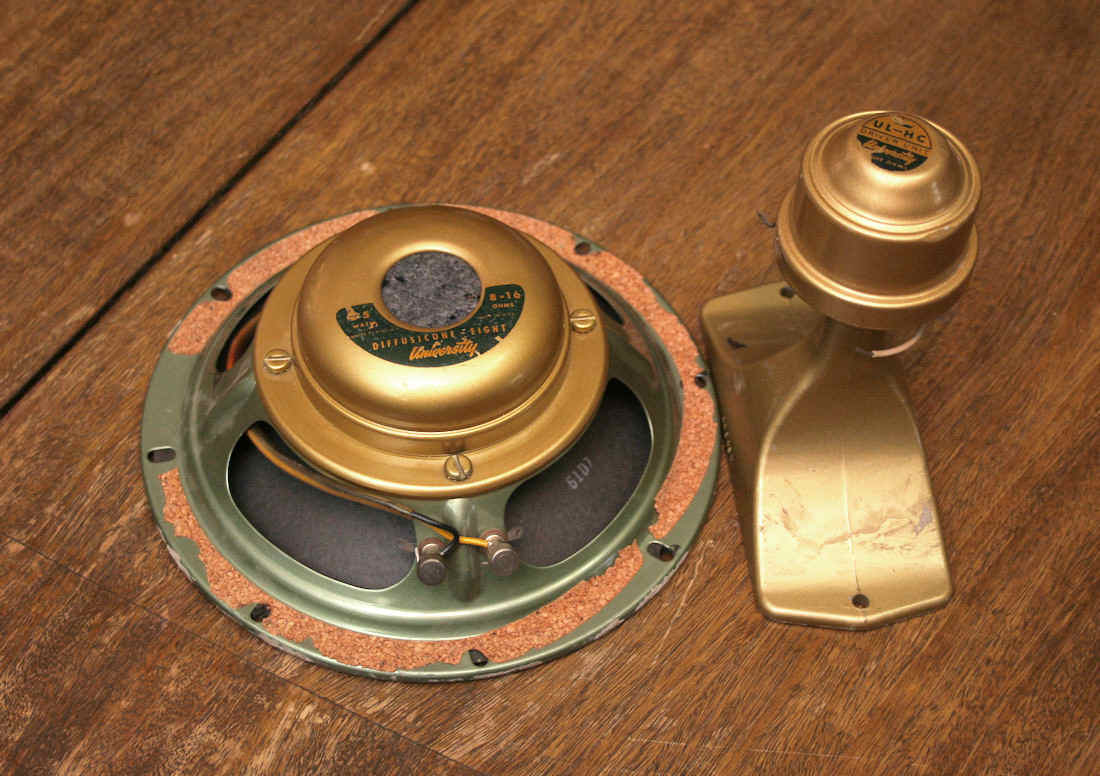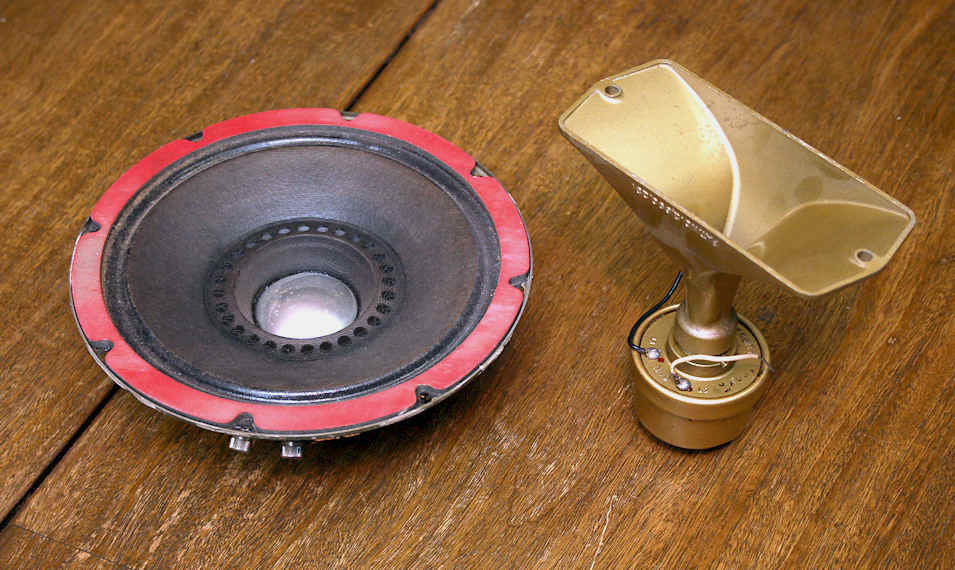C15HC  DUAL IMPEDANCE HIGH COMPLIANCE WOOFER

Two views of the C-15HC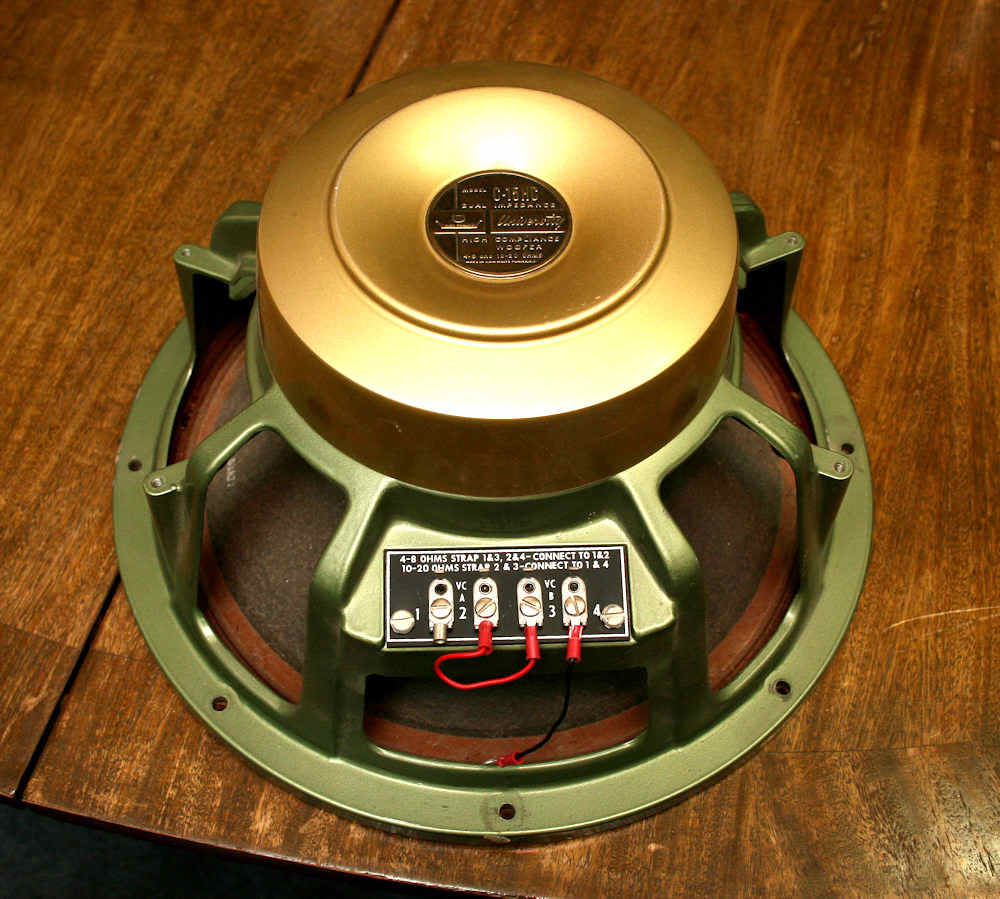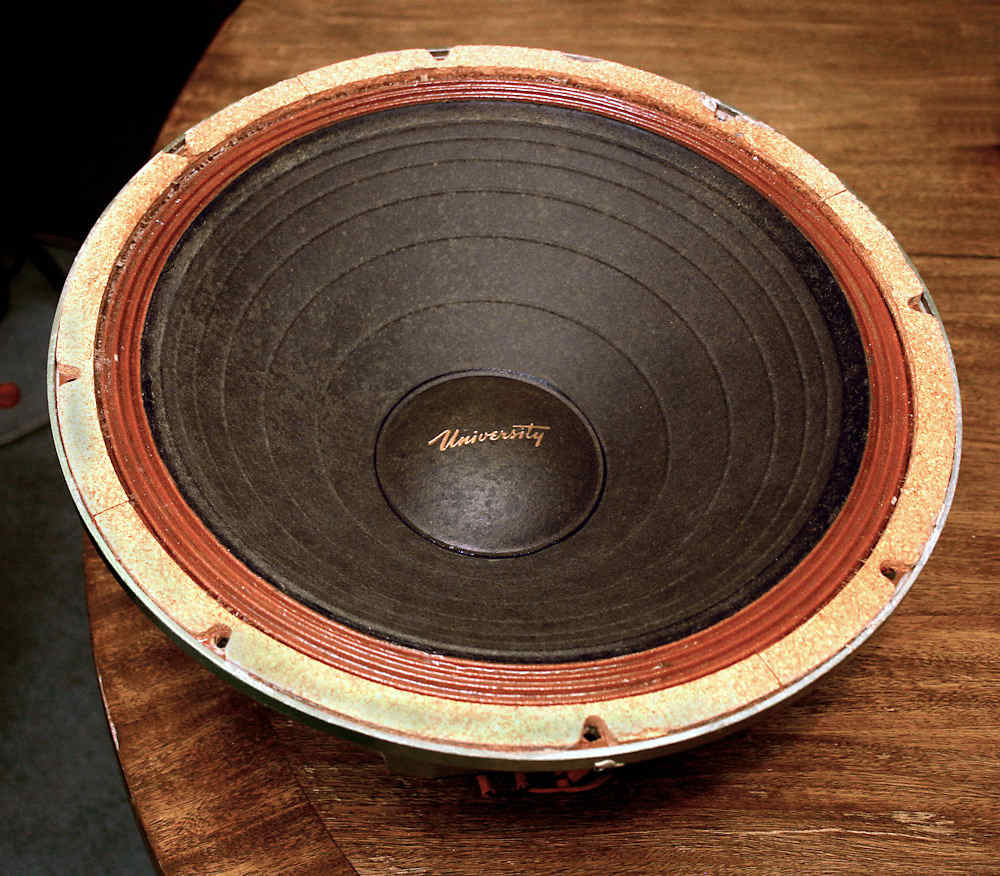Impedance curves.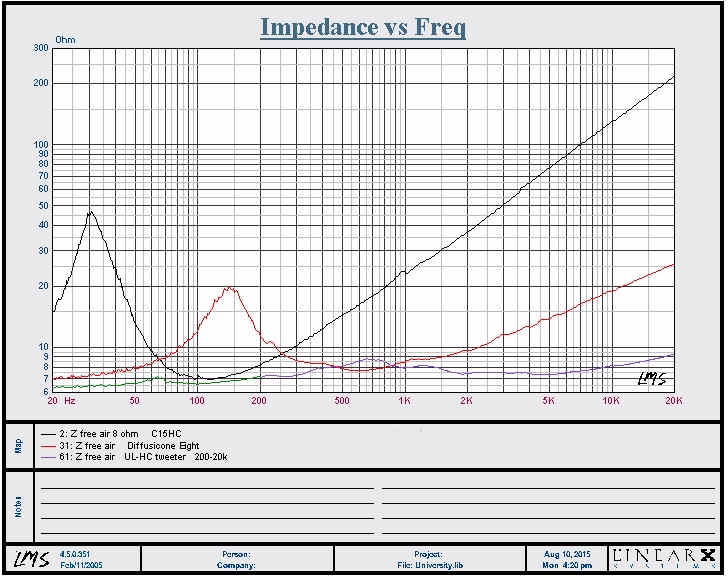Near field response curves. #7  green: the longest one is the 15 incher.   It can be seen to be quite good to 500 hz. #36  black: the 8" diffusicone.  The sweep was stopped at 500 hz as the gating becomes erratic at close to 400 hz due to the wavelength approaching the distance of the nearest reflection.  It can be seen to be pretty decent to about 4 khz although it could be pushed to 10 khz, depending on one's listening preferences. The horn tweeter (blue) tested here isn't intended or implied to be used in this particular combination.  It's output is rather disappoi8nting but may be due to dust or dirt in the voice coil gap. Taking the unit apart was abandoned due to it's not wanting to co-operate. The red curve is the blue curve increased by 14 dB using the LMS software.  It's response is very similar to the 8" with the exceptions of a much better response above 10khz and to 15 khz. and its being much smoother, not to mention not having a dip between 2khz and 3khz, centered at 2.5khz.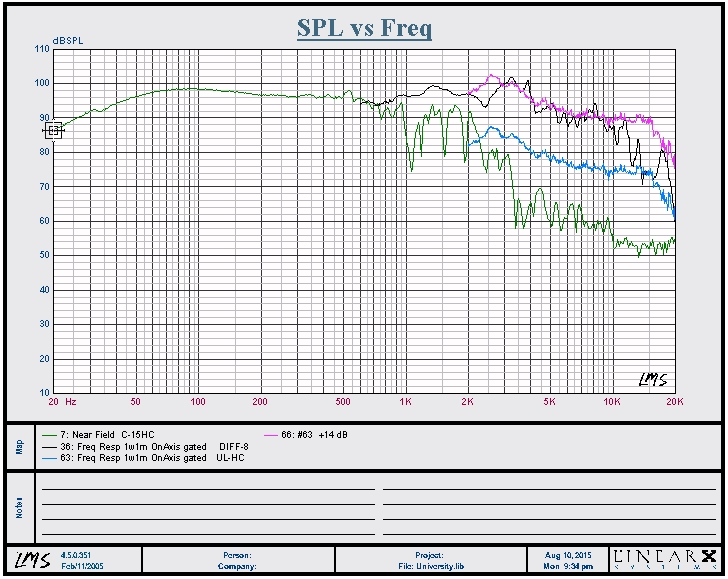Then two upper traces are for the Diffusicone 8, black-on axis and green-30 degrees off axis. The three shorter traces are those of the tweeter.  Blue-on axis; red-30 degrees off axis horizontally and dark blue-30 degrees off axis vertically. From the latter two, the tweeter should be mounted with its laonger dimension horizontal.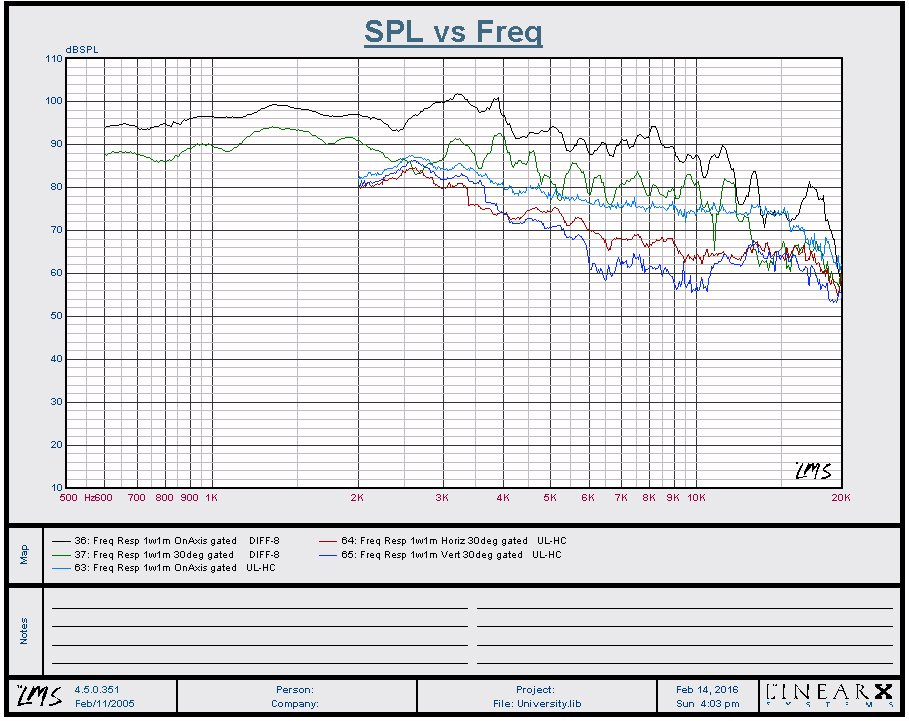RED CURVE Optimum tuning, 9.4 ft^3 Driver Properties Name: C-15HC Type: Standard one-way driver Company: University No. of Drivers = 1 Fs = 30.84 Hz Qms = 3.28 Vas = 242.6 liters Cms = 233.1 µm/N Mms = 114.3 g Rms = 14.88 lb/s Xmax = 5 mm Xmech = 0.394 in P-Dia = 13 in Sd = 0.0856 sq.m P-Vd = 0.0151 cu.ft Qes = 0.508 Re = 6.19 ohms Le = 3.23 mH Z = 7.428 ohms BL = 16.43 Tm Pe = 100 watts Qts = 0.44 no = 1.339 % 1-W SPL = 93.47 dB 2.83-V SPL = 94.58 dB ----------------------------------------- Box Properties Name:  Type: Vented Box Shape: Prism, square (optimum) Vb = 9.376 cu.ft Fb = 26.99 Hz QL = 5.177 F3 = 32.83 Hz Fill = normal No. of Vents = 2 Vent shape = round Vent ends = one flush Dv = 3.02 in Lv = 2.102 in Vented Enclosure, bass reflex   These curves show the level normalized to 0 dB.  The high end rolloff is due to voice coil inductive reactance and the low end rolloff is due primarily to the excursion and thermal limitations as well as cabinet loading and tuning.   The vent tuning for these alignments are to allow maximum excursion to Xmax, sometimes referred to as maximum power vent. These are the curves for the particular unit I have.  The annulus has been softened with acetone and lacquer thinner.  It was treated several years ago but that data was lost due to a drive failure. The next set of curves are those of the pre-treated annulus. If you have a way to measure the fundamental (free air) resonance of your C-15HC, the alignments here can be used by selecting the one with the closest Fs to your unit.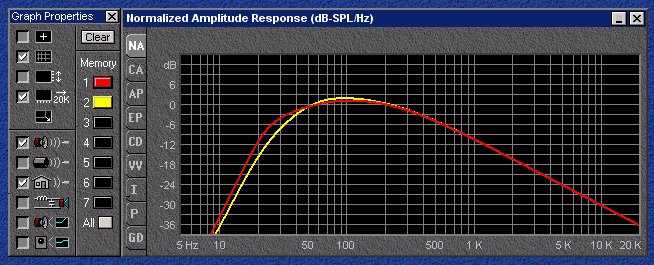YELLOW CURVE Practical tuning, 5 ft^3 Driver Properties Name: C-15HC Type: Standard one-way driver Company: University No. of Drivers = 1 Fs = 30.84 Hz Qms = 3.28 Vas = 242.6 liters Cms = 233.1 µm/N Mms = 114.3 g Rms = 14.88 lb/s Xmax = 5 mm Xmech = 0.394 in P-Dia = 13 in Sd = 0.0856 sq.m P-Vd = 0.0151 cu.ft Qes = 0.508 Re = 6.19 ohms Le = 3.23 mH Z = 7.428 ohms BL = 16.43 Tm Pe = 100 watts Qts = 0.44 no = 1.339 % 1-W SPL = 93.47 dB 2.83-V SPL = 94.58 dB ----------------------------------------- Box Properties Name:  Type: Vented Box Shape: Prism, square Vb = 5 cu.ft Fb = 31.97 Hz QL = 6.788 F3 = 39.06 Hz Fill = normal No. of Vents = 2 Vent shape = round Vent ends = one flush Dv = 3 in Lv = 3.943 in

 RED CURVE Optimum tuning, 16.4 ft^3 Driver Properties Name: C-15HC stiff Type: Standard one-way driver Company: University No. of Drivers = 1 Fs = 39.77 Hz Qms = 3.912 Vas = 113 liters Cms = 108.6 µm/N Mms = 147.5 g Rms = 20.78 lb/s Xmax = 5 mm Xmech = 0.394 in P-Dia = 13 in Sd = 0.0856 sq.m P-Vd = 0.0151 cu.ft Qes = 1.048 Re = 6.19 ohms Le = 3.23 mH Z = 7.428 ohms BL = 14.76 Tm Pe = 100 watts Qts = 0.827 no = 0.648 % 1-W SPL = 90.32 dB 2.83-V SPL = 91.43 dB ----------------------------------------- Box Properties Name:  Type: Vented Box Shape: Prism, square (optimum) Vb = 16.42 cu.ft Fb = 28.94 Hz QL = 5 F3 = 24.59 Hz Fill = normal No. of Vents = 4 Vent shape = round Vent ends = one flush Dv = 3 in Lv = 1.338 in Vented Enclosure, bass reflex   The untreated annulus.   The fundamental resonance, Fs was reduced from 39.77 hz to 30.84 hz. The minimum cabinet volume was chosen at 9 ft^3; at 5 ft^3, the peak at 60 hz was about 5 dB higher which would be very objectionable.  Even this 5 dB peak can be objectionable with male voice and cello, depending on one's preference.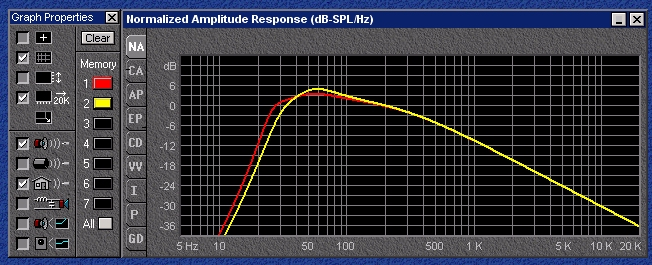YELLOW CURVE Practical tuning, 9 ft^3 Driver Properties Name: C-15HC stiff Type: Standard one-way driver Company: University No. of Drivers = 1 Fs = 39.77 Hz Qms = 3.912 Vas = 113 liters Cms = 108.6 µm/N Mms = 147.5 g Rms = 20.78 lb/s Xmax = 5 mm Xmech = 0.394 in P-Dia = 13 in Sd = 0.0856 sq.m P-Vd = 0.0151 cu.ft Qes = 1.048 Re = 6.19 ohms Le = 3.23 mH Z = 7.428 ohms BL = 14.76 Tm Pe = 100 watts Qts = 0.827 no = 0.648 % 1-W SPL = 90.32 dB 2.83-V SPL = 91.43 dB ----------------------------------------- Box Properties Name:  Type: Vented Box Shape: Prism, square Vb = 9 cu.ft Fb = 36.68 Hz QL = 6.214 F3 = 29.11 Hz Fill = normal No. of Vents = 4 Vent shape = round Vent ends = one flush Dv = 2.444 in Lv = 0.75 in

 TREATED   Driver Properties Name: C-15HC Type: Standard one-way driver Company: University No. of Drivers = 1 Fs = 30.84 Hz Qms = 3.28 Vas = 242.6 liters Cms = 233.1 µm/N Mms = 114.3 g Rms = 14.88 lb/s Xmax = 5 mm Xmech = 0.394 in P-Dia = 13 in Sd = 0.0856 sq.m P-Vd = 0.0151 cu.ft Qes = 0.508 Re = 6.19 ohms Le = 3.23 mH Z = 7.428 ohms BL = 16.43 Tm Pe = 100 watts Qts = 0.44 no = 1.339 % 1-W SPL = 93.47 dB 2.83-V SPL = 94.58 dB ----------------------------------------- Box Properties Name:  Type: Closed Box Shape: Prism, square Vb = 3.45 cu.ft Qtc = 0.614 QL = 15.81 F3 = 58.76 Hz Fill = heavy Sealed Enclosure A comparison between the untreated and treated units in a sealed enclosure.  There isn't much difference between them but the response is 6 dB down at 50 hz.  Admittedly, the sealed box will give a better transient response and a bass boost will bring up the bottom end although that can be risky with such an old speaker.  The adhesive holding the voice coil winding and layers together have also aged by some 55 years.  I had a 60 year old Wharfedale aluminum voice coil come apart with less than 40 watts.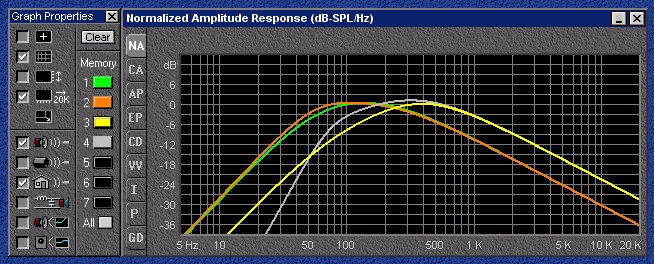Yellow = closed 4.8 ft^3       grey = vented  1.6 ft^3 both are optimized volumes These two curves are those of an untreated C15W with an Fs of 70 hz.  The annulus was very hard and stiff, due to age+.  It's difficult to tell it apart from the C-15HC without looking at the label on the magnet cover.  The speaker, when new, had to have had an Fs of 28 hz or lower, as would have been the case with the C-15HC.  Both units have dual voice coils that can be wired for 4 to 8 ohms or 10 to 20 ohms. NOT-TREATED   Driver Properties Name: C-15HC stiff Type: Standard one-way driver Company: University No. of Drivers = 1 Fs = 39.77 Hz Qms = 3.912 Vas = 113 liters Cms = 108.6 µm/N Mms = 147.5 g Rms = 20.78 lb/s Xmax = 5 mm Xmech = 0.394 in P-Dia = 13 in Sd = 0.0856 sq.m P-Vd = 0.0151 cu.ft Qes = 1.048 Re = 6.19 ohms Le = 3.23 mH Z = 7.428 ohms BL = 14.76 Tm Pe = 100 watts Qts = 0.827 no = 0.648 % 1-W SPL = 90.32 dB 2.83-V SPL = 91.43 dB ----------------------------------------- Box Properties Name:  Type: Closed Box Shape: Prism, square (optimum) Vb = 4.806 cu.ft Qtc = 0.736 QL = 5 F3 = 48.65 Hz Fill = heavy

Back to the loudspeaker main page